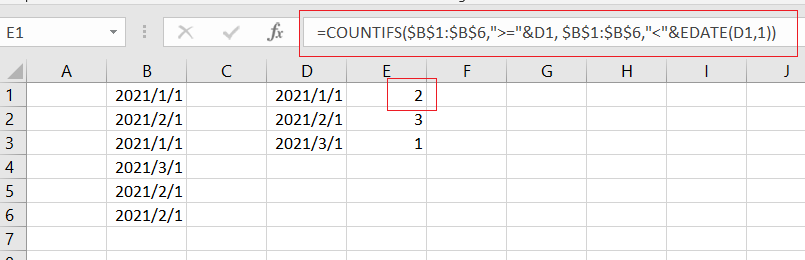# How to Create a Summary Count by Month with COUNTIFS in Excel

This post will guide you how to create a summary count by month with COUNTIFS function in Excel 2013/2016 or Excel office 365. You can use the COUNTIF function to count cells with a given criteria in excel. this guide will show you the simple formula used to create a summary count by month with COUNTIFS function and the EDATE function with two criteria in Excel.

## Summary Count by Month with COUNTIFS

Assuming you have a list of data in range B1:B6 that contain date values, and you want to get a total count per month in your summary table(D1:D3) in your worksheet, and you can use a formula based on the COUNTIFS function to generate a total count per month. Like this:

``=COUNTIFS(\$B\$1:\$B\$6,">="&D1, \$B\$1:\$B\$6,"<"&EDATE(D1,1)) ``Let’s See How This Formula works:

The COUNTIFS function can be used to count the number of cells that meet multiple conditions or criteria. And the Cell D1 is a date for the first of each month in 2021. And if you want to generate a total count per month, and you need to provide criteria that will filter all the date values that appear in each month in range B1:B6.

You still need to build criteria that is a second date can be created with the EDATE function. It means that dates must be greater than or equal to the data in cell D1 or less than the data in D1 plus one month (get it with EDATE function).

### Related Functions

• Excel COUNTIFS function
The Excel COUNTIFS function returns the count of cells in a range that meet one or more criteria. The syntax of the COUNTIFS function is as below:= COUNTIFS(criteria_range1, criteria1, [criteria_range2, criteria2]…)…
• Excel COUNTIF function
The Excel COUNTIF function will count the number of cells in a range that meet a given criteria. This function can be used to count the different kinds of cells with number, date, text values, blank, non-blanks, or containing specific characters.etc.= COUNTIF (range, criteria)…
• Excel EDATE function
TThe Excel EDATE function returns the serial number that represents the date that is a specified number of months before or after a specified date.The syntax of the EDATE function is as below:=EDATE (start_date, months)…